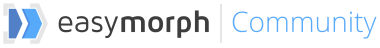# Iterate same row in table a known number of times

Hi - I am trying to extract data from some extremely large tables (10’s of millions of rows) which are too large for EM to handle in one pass so I am trying to chunk the tables. My issue is that I don’t have an element that works in an efficient enough manner. My thought now is to attempt something like this:

1. Get row count of table
2. calculate the number of iterations needed for efficient processing (right now about 1M rows per iteration) round((row_count/1000000) + 1,0) - table has 1M+ rows would come out to 2 iterations.
3. iterate against the table for that number of times - iteration has sql where clause that would use a modulus calc to determine which batch of data to select (first iteration would grab 1M rows, next would grab the rest).

What I am struggling with is how to define the loop - do I use REPEAT or something else?

Thanks,

Keith

I wouldn’t use “Repeat” here because it’s more complex. A regular iteration would suffice and is easier to arrange.

The trick here is to partition the big table by a field, rather than by row count.

Try to find a field that can be used to partition the table. For instance, date or year, or product group. Partitions (i.e. group of rows with the same partition value, e.g. date) should have roughly similar number of rows (e.g. between 100K and 1mln).

If a field like that exists, then build your iteration as follows:

1. Select and import all distinct values of the field. For instance, for [Date] it will be all unique dates in the table.
2. Iterate across the list, by calling for each distinct date a module that …
3. … has date as a parameter, imports from the database only a subset of rows for one date (which is specified by the parameter), and processes the rows in one chunk.

Note that both visual query builder and custom SQL allow using a parameter for filtering.

If no field like that exists, then partitioning should be done by rows and chunk offsets should be calculated prior to iteration. This can be done when the total row count is known. If not, then we will have to resort to the “Repeat” action.

Let me know if you need an example.

Hi Dmitry,

My issue is that I don’t have an element that will allow me to do that in an efficient manner. If I use a date element then I end up with 1800 iterations or if I use another element then I end up with 38K iterations. I am up against the fact that the most efficient manner to extract is by grabbing the data in batches of 1M rows each.

Keith

Keith,

If you can get the total row count beforehand, then you can use the batch offset calculator below for pre-calculating batch offsets and iteration. Insert the offset and batch size in the SQL query in each iteration. In the 1st iteration there is no offset, apparently.

batch-calculator.morph (2.6 KB)

Thanks - this helps. Performance is still an issue though.